0
53

# Quantitative Reasoning Examples and Solutions For Pry 3, 4 & 5

Quantitative Reasoning is a measures a person’s ability to use mathematical or analytical skills in order to solve problems.

Here, I will be solving some examples on quantitative reasoning for primary 3, 4, and 5 pupils.

Example 1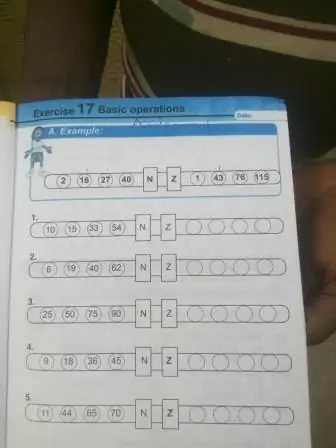Solution

One good thing about quantitative reasoning is that it helps you to think deeply in order to generate the right answer.

The technique used in the above example follow this pattern;

(2*3) – 5 = 1

(16*3) – 5 = 43

(27*3) – 5 = 76

(40*3) – 5 = 115

Use this format to solve the remaining question

(10*3) – 5 = 25

(15*3) – 5 = 40

(33*3) – 5 = 94

(54*3) – 5 = 157

Example 2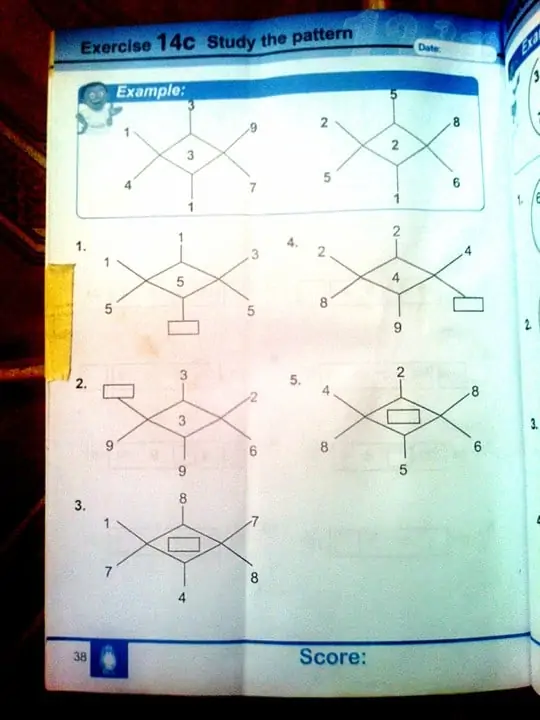Solution

The format to solve this particular question is discussed below;

139 * 3 = 417

258 * 2 = 516

In order to solve the first question,

113 * 5 = 565

Therefore, 6 will be in the box

Example 3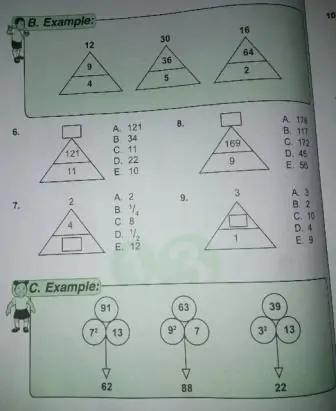Solution

(9*4) divided by square root of 9 = 36/3 = 12

(36*5) / square root of 36 = 180/6 = 30

(64*2)/ square root of 64 = 128/8 = 16

Example 4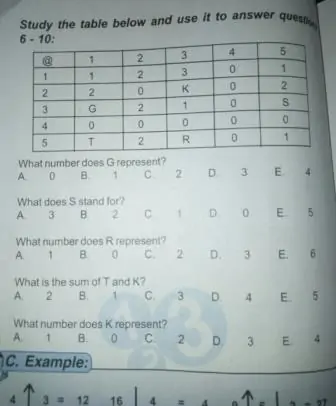Solution

The numbers in COLUMN must be the same in the ROW

For ROW 1, the numbers are 1, 2, 3, 0, 1

For COLUMN 1, the numbers are, 1, 2, G, 0, T

The G is 3

The T is 1

So use the same method for the rest.

READ HERE  Examples Of Corresponding Angles

Example 5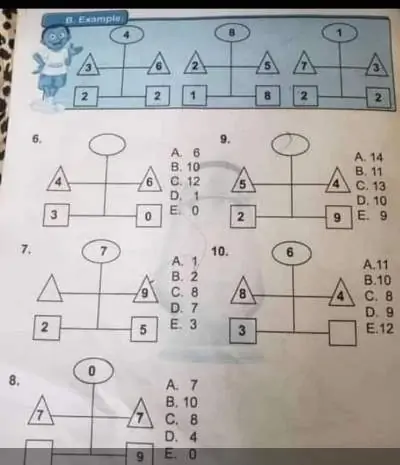Solution

The first sample question: take the 2 and 2 in the square boxes to be 22, multiply the 3 and 6 in the triangle boxes together. then subtract i.e. 22-(6*3) = 4 in the circular box

2nd sample: 18-(2*5) = 8

sample 3: 22-(7*3) = 1

Use this approach to solve question 6-10 of the diagram

Example 6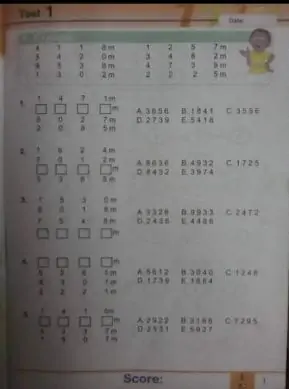Solution

From the samples given, if you add the first two columns together, it will give the third column.

1st example, 4118 + 5420 = 9538

2nd example, 1257 + 3482 = 4739

Also, if you subtract the first column from the second column, it will give you the fourth column.

sample 1, 5420 – 4118 = 1302

sample 2, 3482 – 1257 = 2225

Use this same method to solve question 1-5 of the diagram

Example 7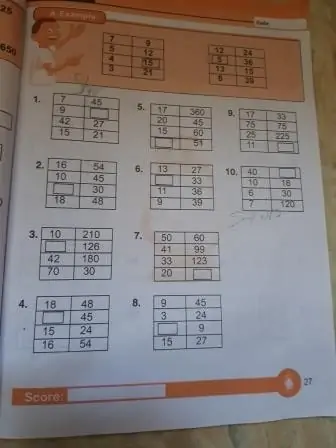Solution

If you look at the first example, add the left hand side together (7+5+4+3 = 19) and the right hand side (9+12+15+21 =57). So if you multiply the sum of the left hand side which is 19 x 3 you will get the sum of the right hand side which is 57

The same for the second example , left hand side 12+5+13+8 = 38, the right hand side is 24+36+15+39 = 114. if you multiply the 38 x 3 = 114

In summary if you add the numbers in the left hand side and multiply by 3, you will get the sum of the numbers on the right hand side.

READ HERE  Variation In Mathematics Types And Examples

Example 8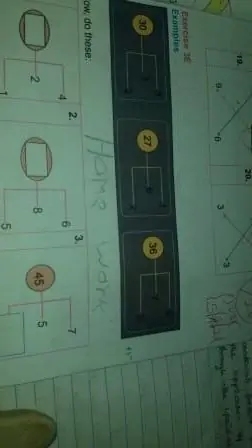solution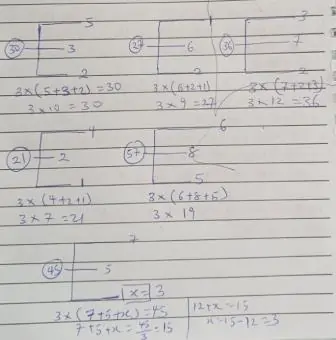Example 9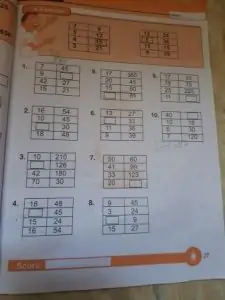Solution

(34/2) + 6 = 23

(49/2) + 6 = 30 1/2

(62/2) + 6 = 37

(76/2) + 6 = 44

The above format should be used to solve the problem.
Example 10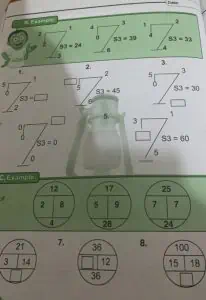Solution

For the first sample, the format is

(2+2+1+3)*3=24

(0+4+3+6)*3 =39

(4+1+2+4)*3=33

You add all the numbers at the edge of the diagram and multiply by 3

For the second sample,

2*8=12+4=16

5*9=17+28=45

7*7=25+24=49

Example 11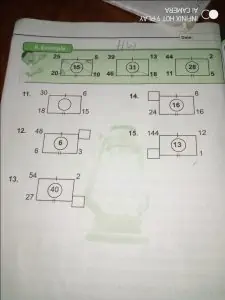From the example, 25/5 = 5, 20-10 = 10

Then add the two answers 5+10 = 15 that is the number in the middle

For the second one, 39/13 = 3, 46-18 = 28, so 28+3 = 31

For the third one, 44/2 = 23, 11-6 = 5, so 23+5 = 28

Example 12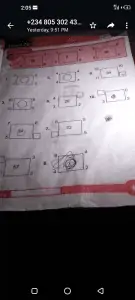For sample 1, (2^2 + 5^2) -(3^2 + 2^2) = 16

(4 +25) – (9+4) = 16

29-13 = 16

For sample 2, (3^2 + 1^2) – (1^2 + 2^2) = 5

(9 + 1) – (1 + 4) = 5

10 – 5 = 5

For sample 3, (5^2 + 5^2) – (4^2 + 2^2) = 30

(25 + 25) – (16 + 4) = 30

50 – 20 = 30

So, you can use the above approach to solve the remaining questions.